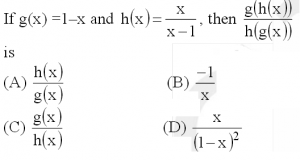Related Articles
GATE | GATE-CS-2015 (Set 1) | Question 65
• Last Updated : 19 Feb, 2015(A) a
(B) b
(C) c
(D) d

Explanation:

```g(h(x)) = g(x/(x-1)) = 1 - x/(x-1) = -1/(x-1)

h(g(x)) = h(1-x) = (1-x)/((1-x) - 1) = -(1-x)/x

g(h(x)) / h(g(x))  = [-1/(x-1)] / [-(1-x)/x]
= -x/(x-1)2

-x/(x-1)2 is same as h(x) / g(x) ```My Personal Notes arrow_drop_up
Recommended Articles
Page :